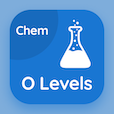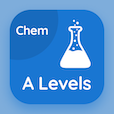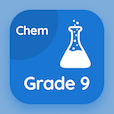Online O Level Courses

O Level Chemistry Practice Tests

O Level Chemistry Tests

The Book Chemical Equations Multiple Choice Questions (MCQ Quiz) PDF, Chemical Equations MCQs download to study online o level chemistry courses. Practice Salts and Identification of Ions and Gases Multiple Choice Questions and Answers (MCQs), Chemical Equations quiz answers PDF for ACT practice test. The eBook Chemical Equations MCQ App Download: salts & identification of ions & gases test prep for online college classes.

The MCQ: Consider the equation: Ca+2 (aq) + 2OH- (aq) → Ca(OH)2 (s). The precipitate of calcium hydroxide will be of PDF, Chemical Equations App Download (Free) with green color, blue color, brown color, and white color choices for ACT practice test. Study chemical equations quiz questions, download Google eBook (Free Sample) for SAT prep classes.

## Chemistry: Chemical Equations MCQs

MCQ: Consider the equation: Ca+2 (aq) + 2OH- (aq) → Ca(OH)2 (s). The precipitate of calcium hydroxide will be of

A) green color
B) blue color
C) brown color
D) white color

MCQ: Consider the equation: Cu+2(aq) + 2OH-(aq) → Cu(OH)2(s). The precipitate of Copper Hydroxide (Cu(OH)2) will be of

A) green color
B) blue color
C) brown color
D) white color

MCQ: Consider the equation: Pb+2 (aq) + 2OH- (aq) → Pb(OH)2 (s). The precipitate of lead (II) hydroxide will be of

A) green color
B) blue color
C) brown color
D) white color

MCQ: In writing chemical equations, the inclusion of state symbols shall be done while the

A) correct chemical formula of reactants and products are written
B) equation is being balanced to fulfill the law of conservation of mass
C) equation has been balanced
D) chemical formulae of products and reactants have been changed to bring about quick balancing

MCQ: Consider Calcium Carbonate (CaCO3) and Hydrochloric Acid (HCl) as reactants, molecules of Hydrochloric Acid (HCl) required to balance the equation will be

A) 1
B) 2
C) 3
D) 4

### Practice Tests: O Level Chemistry Exam Prep

Download O Level Chemistry Quiz App, A level Chemistry MCQ App, and 9th Grade Chemistry MCQs App to install for Android & iOS devices. These Apps include complete analytics of real time attempts with interactive assessments. Download Play Store & App Store Apps & Enjoy 100% functionality with subscriptions!